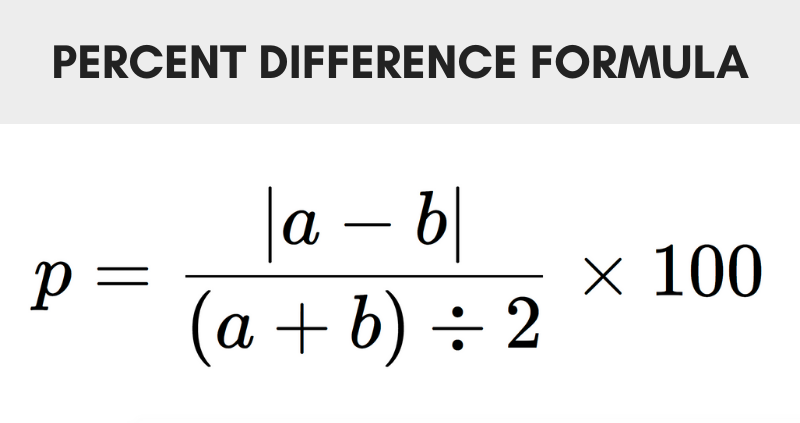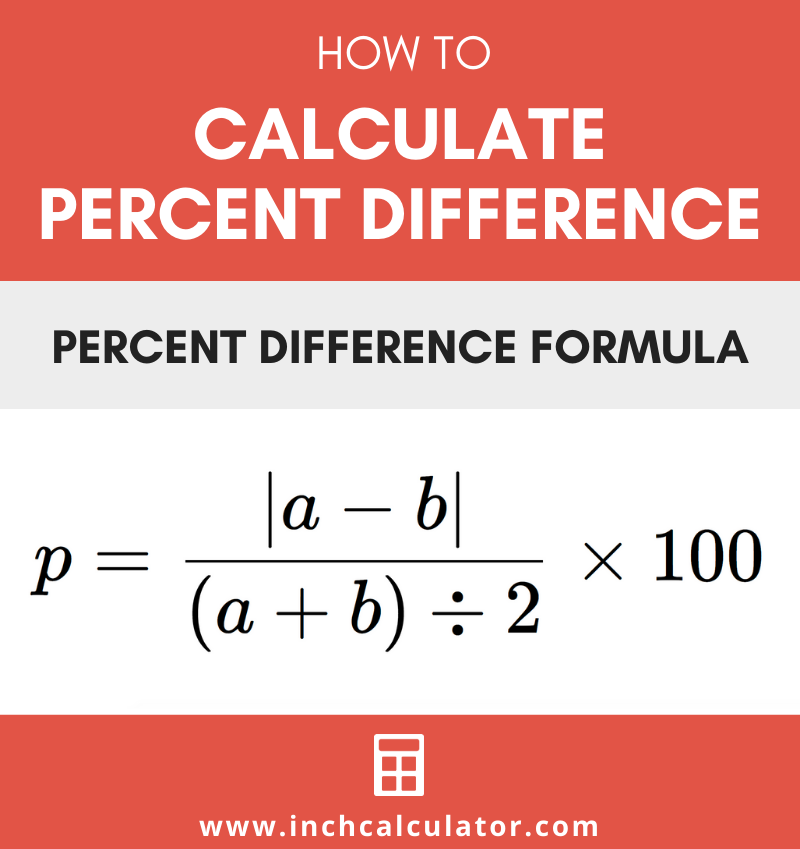# Percent Difference Calculator – with Step-by-Step Guide

Calculate the percent difference between two numbers by entering them below, and we’ll also show the steps to solve it.

## Percent Difference:

Difference between 75 and 100
28.571%

### Steps to Solve Percent Difference

p=|a - b| / (a + b) ÷ 2×100
Substitute values in the equation
p=|75 - 100| / (75 + 100) ÷ 2×100
Simplify and solve
p=|-25| / (175) ÷ 2×100
p=25 / 87.5×100
p=0.28571428571429×100
p=28.571428571429
Learn how we calculated this below

## How to Calculate Percent Difference

Percent difference is a comparison of two numbers of the same measurement. Specifically, it is the difference between two numbers expressed as a percentage.

The percent difference between two values is equal to their difference divided by their average.

So, percent difference can be found by first finding the difference between the numbers, then finding the average of the numbers, and then dividing. Finally, multiply by 100 to get a percentage.

### Percent Difference Formula

The formula for percent difference is:

p = |a – b| / (a + b) ÷ 2 × 100

Thus, percent difference p is equal to the absolute value of a minus b, divided by a plus b divided by 2, times 100.### Steps to Find Percent Difference

The difference can be solved in three easy steps.

#### Step One: Find the Difference

The first step in solving the percent difference is to find the difference between the values. This is as simple as subtracting one value from the other.

The key is to use the absolute value of the difference, which just means to drop the negative sign in front of the number if there is one.

#### Step Two: Find the Average

The second step in calculating the percent difference is to find the average of the values. To do this, add the values together to get the sum, then divide by 2; this is the average.

#### Step Three: Divide the Difference by the Average

The next step is to divide the difference of the values by the average. This is the percent difference expressed as a decimal or fraction.

#### Step Four: Convert to a Percentage

To express the difference as a percentage, simply multiply by 100.

Example: Find the percent difference of 25 and 48

p = |25 – 48| / (25 + 48) ÷ 2 × 100
p = |-23| / 73 ÷ 2 × 100
p = 23 / 36.5 × 100
p = 63.014%

## Percent Difference vs. Percent Change

It’s important to note that percent difference is not the same thing as percent change.

As stated above, the difference is the difference between two numbers expressed as a percentage. Percent change is the change from one value to another value.

If you’re actually trying to solve the amount of change from one value to another, try our percent change calculator.

Of course, our percentage calculator has a ton of tools for calculating percentage that you might also be interested in.